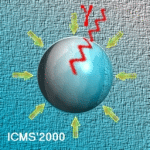# ICMS'2000

The International Conference on Multiphase Systems, ICMS'2000
Ufa, RUSSIA, June 15-17, 2000

Secondary Bjerknes Forces and the Phenomenon of Acoustic Streamers

Pelekasis N. A., Gaki A., Tsamopoulos J. A.

Laboratory of Computational Fluid Dynamics, Department of Chemical Engineering, University of Patras, Patras, GREECE

Doinikov A.

Institute of Nuclear Problems, Byelorussia State University, Minsk, BELARUS

Cavitating bubbles in liquid solutions that are insonated at very high amplitude are often seen to form filamentary structures where each one of them is moving rapidly, while the distance between them remains constant and much larger than their average radius. These structures are called acoustic streamers and the mechanism of their formation is not fully understood, especially since the bubbles that form them are normally small, <10\mu m, and they are driven below resonance, \omega_f<\omega_{0i}; \omega_f, \omega_{0i}, denote the forcing frequency and the fundamental frequencies of the two bubbles, respectively. In the present study the translational velocities of two spherical air bubbles that are oscillating in water in response to a large disturbance in the static pressure field, are calculated. The two bubbles are assumed to be located far enough so that shape oscillations can be neglected. Viscous effects are accounted for, Re=O(1), due to the small size of the bubbles. In this context the translational velocity, which is a direct measure of the secondary Bjerknes force between the two bubbles, of each bubble is calculated for sound intensities as large as the Blake threshold.

When two bubbles of unequal size with radii in the order of 100 \mu m are subjected to a sound wave with, roughly, half their eigenfrequency and amplitude P_A<0.5 atm their translational velocity is seen to change from attractive to repulsive as P_A increases from 0.05 to 0.1 due to the second harmonic, 2\omega_f, of the forcing frequency which grows as a result of nonlinear interaction. Then, as is well known for secondary Bjerknes forces between two bubbles, when \omega_{01}<\omega_f<\omega_{02} the two bubbles repel each other. However, as the amplitude of sound further increases, P \approx 0.5 atm, the two bubbles are again seen to attract each other due to the growth of even higher harmonics that fall outside the range defined by the eigenfrequencies of the two bubbles.

The case of much smaller bubbles was also examined, radii in the order of 5 \mu m, driven well below resonance, \omega_f=2 \pi x 20 KHz, at very large sound intensities, P_A \approx 1 atm, which is a more realistic representation of prevailing conditions in an acoustic streamer. Thus, it was found that the translational velocities of the two bubbles eventually reach a steady oscillation determined by the forcing frequency. Numerical simulations show that the forces between the two bubbles tend to be attractive except for a narrow region of bubble sizes corresponding to a nonlinear resonance. As the distance between them decreases, the region of repulsion is shifted indicating sign inversion of the force between them. As a presiminary conclusion we note that this mechanism seems to be closer to the dynamic behavior of bubbles in acoustic streamers.